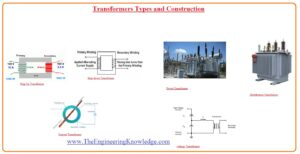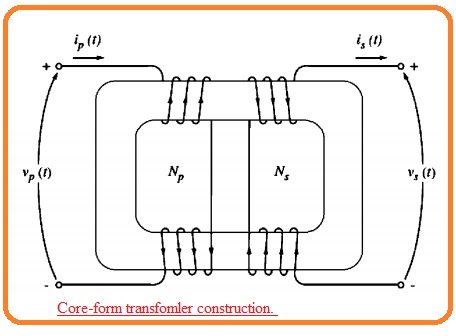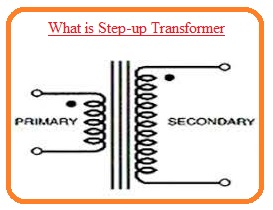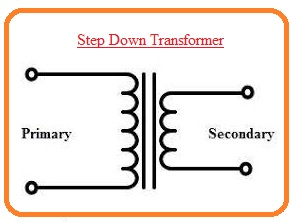Hello, readers welcome to the new post. In this post, we will have a detailed look at the types of Transformers. The transformer is a device that used in an electrical power system to convert voltage level from one to other. Its construction is basic and consists of core and normally two windings first one is called primary that wounded at the input side and other called secondary which is wounded at the secondary side.

According to output get from the transformer, it is configured in step up and step down types. Is the voltage level get at the output is less than the input it called to step down and if the voltage level is high called a step-up transformer. here we cover its different types and their practical uses. So let’s get started with Transformers Types and Construction.

## Transformers Types and Construction

• The main objective of the transformer is to transform ac power from one level to other without varying the value of frequency.
• The transformer also performs other works with varying the voltage level like voltage sampling, current sampling, and transformation of impedance.
• In the case of power transformer, there is 2 category of the core are used. First, it is constructed through the use of rectangle shape steel having lamination material wounded about the core
• This category of structure can seen in the below figure.• The 2nd category constructed with 3 limbs has lamination core wounded at the mid limb.
• this category of construction is called shell type transformer.• The purpose of lamination used in this configuration is to reduce the eddy currents.
• The primary and secondary windings in a practical transformer is wounded at the upper portion and in between there is fewer voltage windings.
• This type of configuration offers 2 main benefits.
• It adjusts the issues of lamination of high voltage coils through the core.
• It helps to reduce the leakage flux that is greater in the case of 2 windings that are isolated through normal distance
• There are many titles given to the power transformer according. The transformer linked to the output terminal of the generator and performing step-up works then this transformer arrangement is known as unit transformer.
• The transformer attached at the second point of transmission line that is performing the step-down work of voltage through transmission to distribution level that this transformer is known as substantial transformation.
• The transformer which gets the output of the substation transformer and changes the voltage level to 110, 208, or 220 is called a distribution transformer.
• There is a special-purpose transformer used in the power system.

### Transformer TypesStep-up Transformer

• This type of transformer used to increase the power level up from one to other
• Its construction is such that there is large number of turns exist at its secondary level ane less turns of windings exist at the input or primary windings.Step Down Transformer

• Contrary to step up transformer this device used to decrease the voltage level at output.
• Its structure is such that there is a large number of turns for windings at the input side and less at output sides. So voltage decreases at output according to value of voltage exist at the input.What is Power Transformer

• The power transformer is used to run larger rating loads like voltage values greater than 33 kilovolts handle through the use of power transformer.
• The physical size of the transformer is larger than other transformers. It normally installed at generation houses to step up voltage to high level since for transmission of voltage to larger distance is necessary to has a large value.

What is Distributiaton Tranformer

• The distribution transformer is also called a service transform that handle a power level of 11 kv, 220, 440.
• It is positioned at the poles so it also named a pole mount transformer. For such a configuration where power lines are buried in the ground then this transform is positioned at the pads created through the use of concrete due to this effect it known as a pad-mounted transformer.
• Its rating is lain the value less than two KVA in some area it has ratings of five thousand KVA.
• There working conditions to all the day so their construction is such that there is very less value of iron losses to make regular working.
• Their operation required ot work at less load than full load so their design is such that they offer the highest value of efficiency at less loads.

Instrument Tranformer

• There are different types of electrical parameters used in ac power systems such as current power, voltage P.F, frequency to finds the value of this parameter transformer used called instruments.
• The main operation of these transformers is to step down the voltage and current of the system so that they can easily measure.
• As the level of these terms in the system is very large so can find at that level there is a need of such a device that can finds the value of these terms easily so voltage value minimized to 110 volts and current five amperes that can be easy finds through normal ammeters and voltmeters.

Potential Transformer

• There is another name of potential transformer is a voltage transformer that used to reduces the voltage at such level that can be easily measured through a normal voltmeter.
• The main benefit of this voltage reduction is made save such devices  like relays and voltmeter that measure this voltage can easily work at that voltage level high voltage can affect their operation
• The operation of this transformer relies at the mutual induction phenomena. It’s primary and secondary has insulation among them but through magnetic flux, they are linked to each other.
• Input or primary side of this device link to the power supply that results in the flux generation in that side and caused the voltage induction in the secondary side according to Faraday law in the secondary side.

That is all about types of transformer. here i mentioned all the types of transformers with the details if you have any further queries and want to get further info ask in the comments Thanks for reading have a good day.